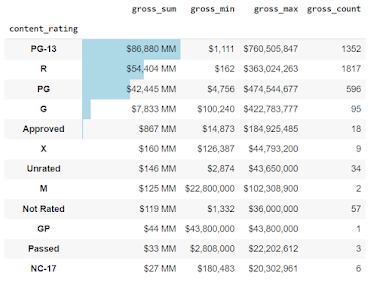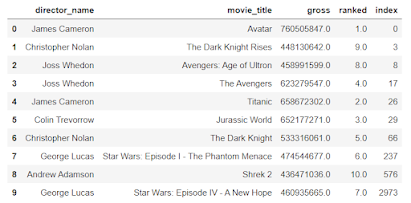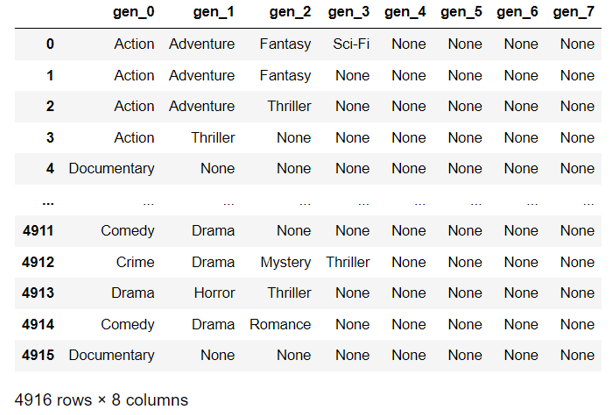## Posts

### DataFrame groupby agg style barThe goal of the article is to investigate the bar function through the style method of Pandas DataFrame.  So, when we work with DataFrames, we can create a visual within a DataFrame.  What does that mean? We can embed bar charts, sparklines, and mini bar charts in the DataFrame.  This can reduce the amount of cognitive load when reviewing a DataFrame.   Google Colab link with all the code To get started, we are going to import the data: import pandas as pd import numpy as np import pandas_profiling as pp loc = 'https://raw.githubusercontent.com/aew5044/Python---Public/main/movie.csv' m = pd.read_csv(loc) pd.set_option('display.max_columns',None) pd.options.display.min_rows = 10 Next, I want to create a new DataFrame that groups by the rating (e.g., "R" "PG-13"), then calculates the total sum, min, max, and total observations for gross.  content = ( m .groupby('content_rating') .agg({'gross':['sum','min&#

### Pandas rankThe goal of this article is to dive into rank in Python's Pandas. We will rank the total gross of a movie and keep the top most-grossing movies. Google Colab Link import pandas as pd import numpy as np loc = 'https://raw.githubusercontent.com/aew5044/Python---Public/main/movie.csv' m = pd.read_csv(loc) pd.set_option('display.max_columns',None) pd.options.display.min_rows = 10 Now that we have our data, we will walk through ranking movies by the variable gross.  Below is my first attempt.  I wanted to chain everything together to be as efficient as possible - which I define as ease of reading and debugging, don't make a bunch of DataFrames between analysis steps, and accuracy, of course.  Below is my first attempt. I wanted the top ten grossing movies from the movies DataFrame.  So, I created a variable called ranked at #1.  I knew I wanted to query in the same chain, so I created a lambda function so the column/variable is usable immediately.   ( m .as

### drop_duplicates DataFrame

Finding unduplicated lists is task number 1 on day 2, so enjoy this quick review on deduplicating a list based on a few paramaters. This narration discusses uses for drop_duplicates. Usually, drop_duplicates is not used in isolation. There are usually steps before the process. The movies DataFrame has a list of movies with director_name and gross. I first want all movies with a gross above 1 MM, and of those movies, the top-grossing moving by the director.  This is how I would approach the task with pandas and chaining.  First, Import the packages and data: Google Colab space with all the executable code import pandas as pd import numpy as np loc = r'C:\....movie.csv' m = pd.read_csv(loc) pd.set_option('display.max_columns',None) pd.options.display.min_rows = 10 Second, apply the desired steps.  At 1, filter out the unnecessary data.  At 2, sort the values by the director's name and gross amount for the movie.  At 3, drop the duplicates by the director

### SplitFor this post, I want to look at an amazingly simple, elegant, and useful method – split. The goal is to look at a column and array out all the data based on a delimiter of our choice.  For example, if I have a column containing all the genres of a movie (action, thriller, adventure, etc.) delimited by a “|” I can use split expand =True to accomplish the task:  When we add the chaining method, we end up with the following code: (m      .genres      .str.split('|', expand=True)      .rename(columns=lambda c: 'gen_'+str(c)) ) This gives us a wonderful DataFrame representing a column for each delimited value. In this example, we see eight columns represented by 0 through 7. I wanted to rename the columns with the prefix "gen_" followed by the numeric index.  There we go, we have our columns split out, ready to be joined back to our original table (if needed).

### Exploratory Data Analysis

Exploratory Data Analysis (EDA) Situation: I’m at a new analyst job, and I’m given access to a new database. What next? Do I wait for a data request?  Do I wait for a random task from my boss? Do I review established reports?  What do I do? I just started a new job in February of 2022. Every day I continually see new datasets, inherit established code, and update old code.  I like to ask open questions: What does each row represent? What are the main “filter” columns? What are the data types? How did the last analyst handle the data? Each list of questions and associated processes are unique to each person and the tasks at hand.  By now, this process is second nature. The purpose of this blog is to learn about Pandas. How  would I approach this in Pandas? Let's dig in! Assumptions, I have access to the table. In this example, m associated with movies as a DataFrame  First, I want to look at everything. The describe method is a method to quickly look at everything in t